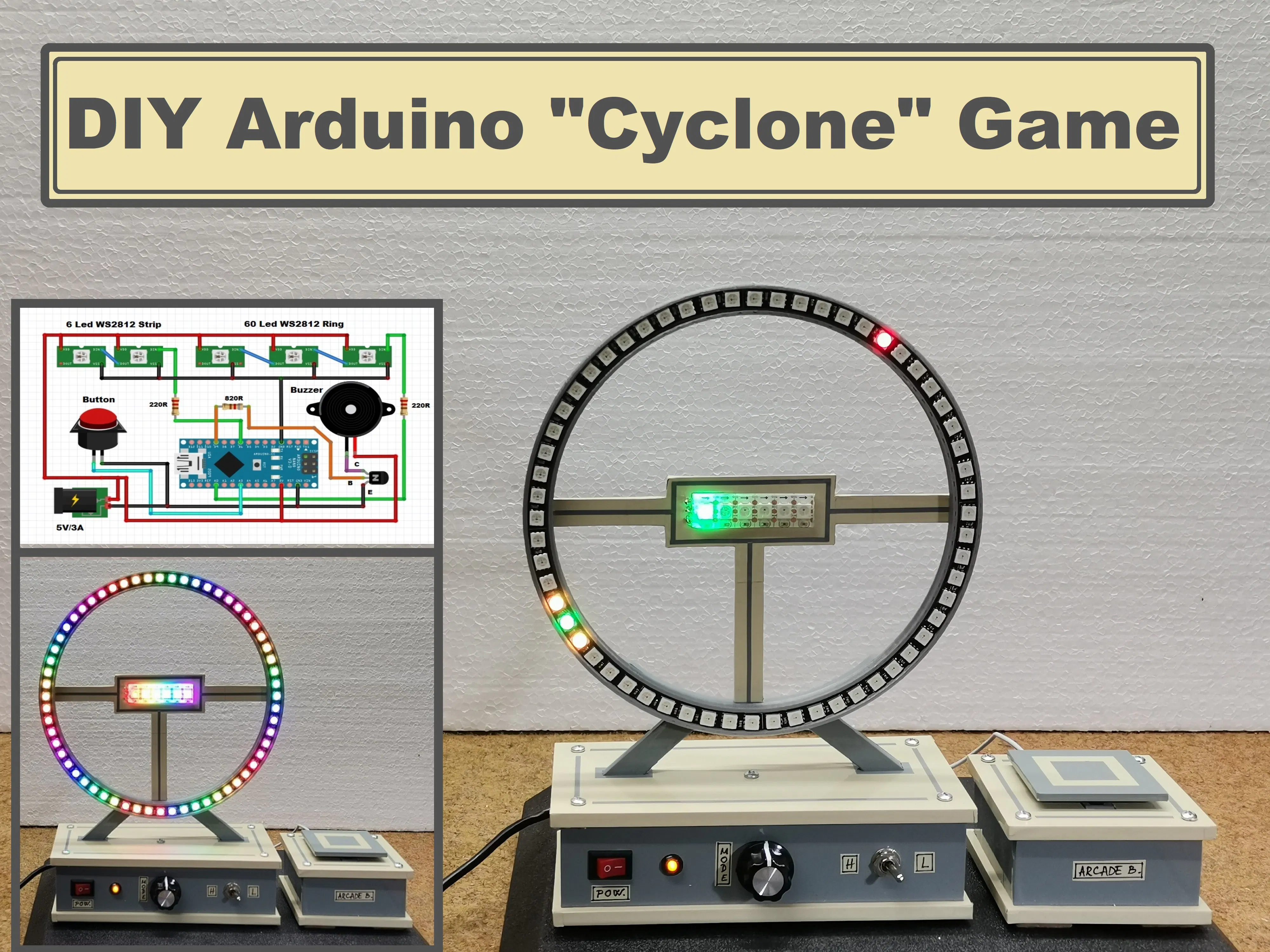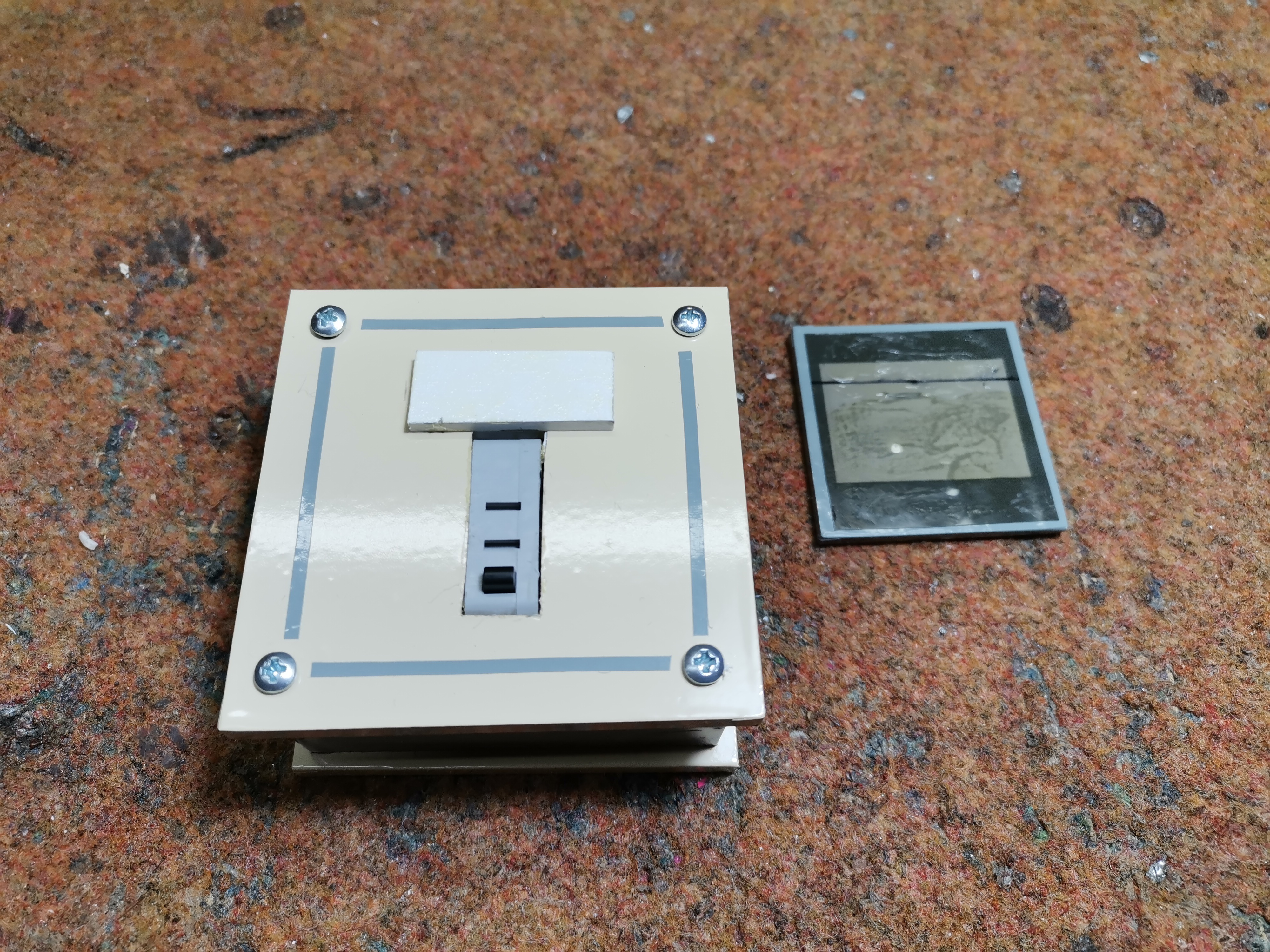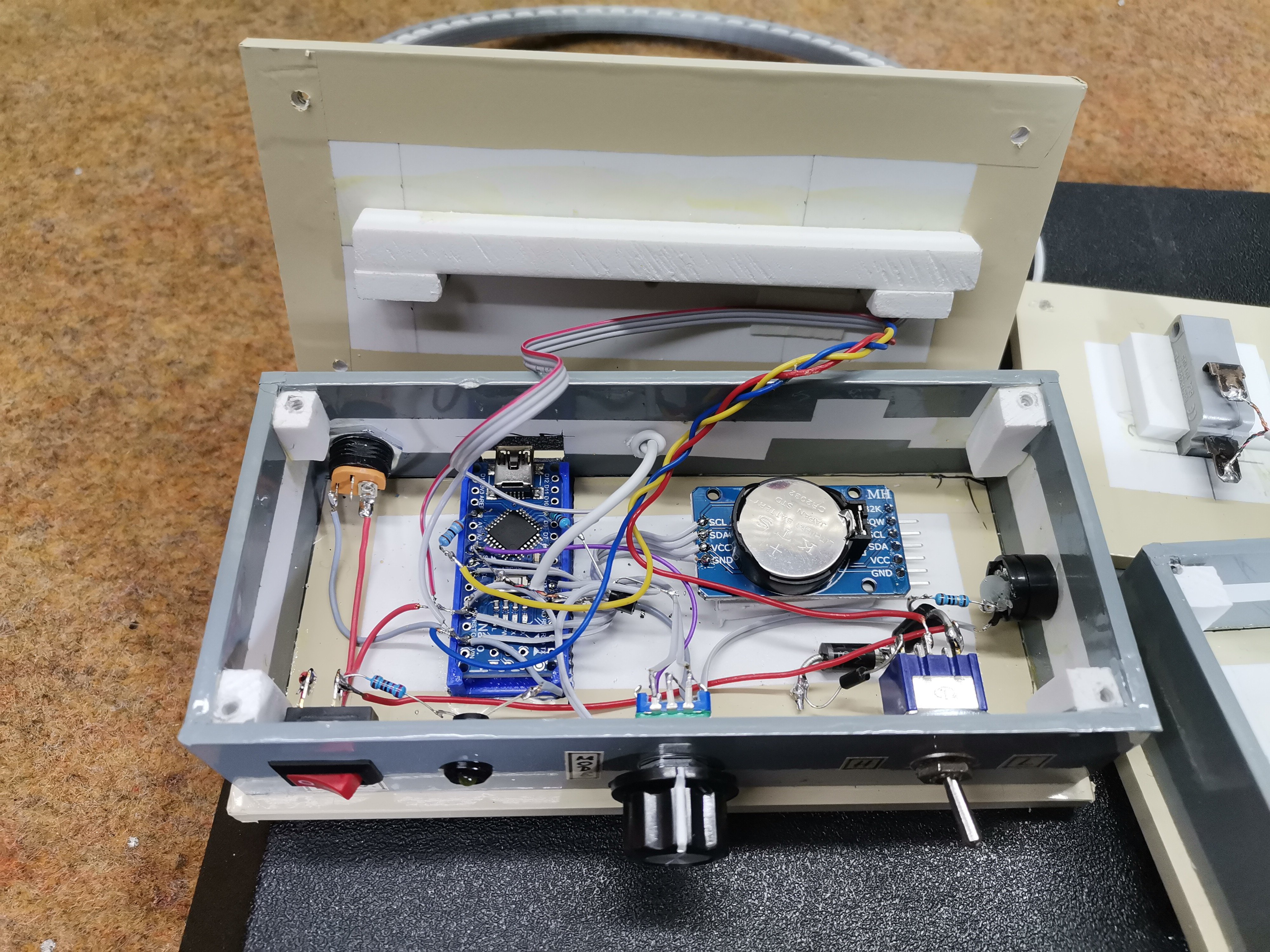Arduino

# DIY Arduino Cyclone Game with WS2812B Led RingMarch 19, 2022 by Mirko Pavleski
ShareBased on the arcade game called Cyclone, where the player tries to stop an led scrolling around a circle at a specific spot

## Hardware

 1 Arduino Nano R3 1 WS2812 Ring with 60 Leds 1 Rotary Encoder with Push-Button 1 Big homemade Arcade button 1 General Purpose Transistor NPN 1 Buzzer 2 Resistor 220 ohm 1 Through Hole Resistor, 820 ohm

## Software

 1 Arduino IDE

## Tools

 1 Soldering iron (generic) 1 Solder Wire, Lead Free

This time I will show you how to make an interesting game that is based on the arcade game called Cyclone, where the player tries to stop an led scrolling around a circle at a specific spot. The goal of the game is to stop the cycling light when it reaches the indicated (red) LED. If successful, the difficulty level will increase. If unsuccessful, the Game will restart.

The ring consists of 60 LEDs (4 Quarter circle neopixels of 15 Leds). The ring holder is made with a 3D printer and you can download the.stl file below. In the middle of the ring is a strip of 6 RGB LEDs that actually represent the six levels. The basic code is taken from oKeeg's Instructable, and I made a few modifications to make the game even more interesting.

• First I reduced the light intensity of the diodes, because in my version of the game the LEDs are directly visible.
• Then, instead of 40, the ring contains 60 LEDs because I used it from one of my previous projects (LED ring clock).
• I also added two more levels so that the game now has a total of 6 levels.
• The next, very important modification is that I added sounds to the game and now it is much more interesting to play.

-Finally, I made a big Arcade button for much more accurate playing.

The game would need to be powered by a Power supply of 5V/3A or more. This is a very simple project and consists only a few components:

- Arduino nano microcontroller

- WS2812 Led ring with 60 RGB leds

- WS2812 Led strip with 6 RGB Leds

- One transistor

- Buzzer

- And big DIY Arcade button

The real time clock module you see in the pictures is not active and is part of my previous project.

We can also use the rotary encoder button left over from the previous project. In this case the device is small and compact, but playing with this button is more difficult.

When turning on the game, all LEDs light up with different colors. Now by pressing the button, the game begins. The goal is to press the button at the moment when the rotating diode is located exactly on the static diodes. In the first two levels, three diodes are static, and in the next levels only one. Also with each next level, the diode speed increases, so it is increasingly difficult to press the button at the required moment. With each completed level, one diode from the horizontal row lights up. There are six levels in total, so this row contains six dodes. If we fail to pass the level, the game starts from the beginning. If you pass all six levels, the game also starts from the beginning.

Finally, the device is built into a suitable box made of PVC board and coated with self-adhesive colored wallpaper.``````#include "FastLED.h"
#define NUM_LEDS 60
#define DATA_PIN A0
#define SCORE_PIN 6
#define SCORE_LEDS 6
#define BRIGHTNESS 55
CRGB leds[NUM_LEDS];
CRGB sleds[NUM_LEDS];

bool reachedEnd = false;
byte gameState = 0;
//byte ledSpeed = 0;
int period = 1000;
unsigned long time_now = 0;
byte Position = 0;
byte level = 0;

const byte ledSpeed = {50, 40, 30, 20, 14, 7};

//Debounce
bool findRandom = false;
byte spot = 0;

void setup() {
// put your setup code here, to run once:
pinMode(A3, INPUT_PULLUP);
Serial.begin(9600);
Serial.println("Reset");
}

void loop() {
// put your main code here, to run repeatedly:
FastLED.setBrightness(BRIGHTNESS );
if (gameState == 0) {
fill_rainbow(leds, NUM_LEDS, 0, 20); //2 = longer gradient strip
fill_rainbow(sleds, SCORE_LEDS, 0, 40); //2 = longer gradient strip

Position = 0;
findRandom = true;
delay(500);
for (byte i = 0; i < NUM_LEDS; i++) {
leds[i].setRGB(0, 0, 0);
delay(40);
FastLED.show();
int thisPitch = map (i, 60, 0, 100, 1500);
tone(9, thisPitch,120);
}
for (byte i = 0; i < SCORE_LEDS; i++) {
sleds[i].setRGB(0, 0, 0);
delay(100);
FastLED.show();
}
gameState = 1;
}
FastLED.show();
}
if (gameState == 1) {
period = ledSpeed;
if (millis() > time_now + period) {
time_now = millis();
if (findRandom) {
spot = random(56) + 3;
findRandom = false;
}
leds[spot - 1].setRGB(255, 140, 0);
leds[spot].setRGB(0, 255, 0);
leds[spot + 1].setRGB(255, 110, 0);
sleds.setRGB(0, 255, 0);
PlayGame(spot - 1, spot + 1);
}
delay(300);
findRandom = false;
if (Position > spot - 1 && Position < spot + 3) {
level = gameState;
gameState = 98;
} else {
gameState = 99;
}
}
}
if (gameState == 2) {
//    period = 320;
period = ledSpeed;
if (millis() > time_now + period) {
time_now = millis();
if (findRandom) {
spot = random(56) + 3;
findRandom = false;
}
leds[spot - 1].setRGB(255, 190, 0);
leds[spot].setRGB(0, 255, 0);
leds[spot + 1].setRGB(255, 190, 0);
sleds.setRGB(255, 255, 0);
PlayGame(spot - 1, spot + 1);
}
delay(300);
if (spot - 1 && Position < spot + 3) {
level = gameState;
gameState = 98;
} else {
gameState = 99;
}
}
}
if (gameState == 3) {
period = ledSpeed;
if (millis() > time_now + period) {
time_now = millis();
if (findRandom) {
spot = random(56) + 3;
findRandom = false;
}
leds[spot].setRGB(0, 255, 0);
sleds.setRGB(255, 50, 0);
PlayGame(spot, spot);
}
delay(300);
if (Position == spot+1) {
level = gameState;
gameState = 98;
} else {
gameState = 99;
}
}
}
if (gameState == 4) {
period = ledSpeed;
if (millis() > time_now + period) {
time_now = millis();
if (findRandom) {
spot = random(56) + 3;
findRandom = false;
}
leds[spot].setRGB(0, 255, 0);
sleds.setRGB(255, 0, 0);
PlayGame(spot, spot);
}
delay(300);
if (Position == spot+1) {
level = gameState;
gameState = 98;
} else {
gameState = 99;
}
}
}

if (gameState == 5) {
period = ledSpeed;
if (millis() > time_now + period) {
time_now = millis();
if (findRandom) {
spot = random(56) + 3;
findRandom = false;
}
leds[spot].setRGB(0, 255, 0);
sleds.setRGB(0, 50, 255);
PlayGame(spot , spot);
}
delay(300);
if (Position == spot+1) {
level = gameState;
gameState = 98;
} else {
gameState = 99;
}
}
}

if (gameState == 6) {
period = ledSpeed;
if (millis() > time_now + period) {
time_now = millis();
if (findRandom) {
spot = random(56) + 3;
findRandom = false;
}
leds[spot].setRGB(0, 255, 0);
sleds.setRGB(0, 150, 255);
PlayGame(spot , spot);
}
delay(300);
if (Position == spot+1) {
level = gameState;
gameState = 98;
} else {
gameState = 99;
}
}
}

if (gameState == 98) {
winner();
}
if (gameState == 99) {
loser();
}
}
void PlayGame(byte bound1, byte bound2) {
leds[Position].setRGB(255, 0, 0);
if (Position < bound1 + 1 || Position > bound2 + 1) {
leds[Position - 1].setRGB(0, 0, 0);
}
FastLED.show();
Position++;
if (Position >= NUM_LEDS) {
leds[Position - 1].setRGB(0, 0, 0);
Position = 0;
}
}

void winner() {
for (byte i = 0; i < 3; i++) {
for (byte j = 0; j < NUM_LEDS; j++) {
leds[j].setRGB(0, 255, 0);
tone(9, 1000, 250);
}
FastLED.show();
delay(500);
clearLEDS();
FastLED.show();
delay(500);

}
findRandom = true;
Position = 0;

gameState = level + 1;
if (gameState > 6) {
gameState = 0;
}
}
void loser() {
for (byte i = 0; i < 3; i++) {
for (byte j = 0; j < NUM_LEDS; j++) {
leds[j].setRGB(255, 0, 0);
tone(9, 200, 250);
}
FastLED.show();
delay(500);
clearLEDS();
FastLED.show();
delay(500);
}
gameState = 0;
}
void clearLEDS() {
for (byte i = 0; i < NUM_LEDS; i++) {
leds[i].setRGB(0, 0, 0);
}
}
void winAll(){

}``````